SLASE48A November   2014  – January 2015

PRODUCTION DATA.

• AAV|144

## 6 Specifications

### 6.1 Absolute Maximum Ratings

over operating free-air temperature range (unless otherwise noted)(1)
MIN MAX UNIT
Supply Voltage Range(2) VDDDAC09, VDDDIG09 –0.3 1.3 V
VDDCLK09 –0.3 1.3 V
VDDT09 –0.3 1.3 V
VDDR18, VDDIO, VDDS18, VQPS18 –0.3 2.45 V
VDDAPLL18, VDDAREF18 –0.3 2.45 V
Terminal Voltage Range(2) RX[7..0]P/N –0.5 VDDT09 + 0.5 V V
SDENB, SCLK, SDIO, SDO, TXENA, ALARM, RESETB, SLEEP, TMS, TCLK, TDI, TDO, TRSTB, TESTMODE, SYNC_N_AB, SYNC_N_CD –0.5 VDDIO18 + 0.5 V V
DACCLKP/N, SYSREFP/N –0.5 VDDAPLL18 + 0.5 V V
SYNCBP/N –0.5 VDDS18 + 0.5 V V
LPF –0.5 VDDAPLL18 + 0.5 V V
IOUTAP/N, IOUTBP/N, IOUTCP/N, IOUTDP/N –0.5 1.0 V V
RBIAS, EXTIO, ATEST –0.5 VDDAREF18 + 0.5 V V
IFORCE, VSENSE –0.5 VDDDIG09 + 0.5 V V
AMUX1, AMUX0 –0.5 VDDT09 + 0.5 V V
Peak input current (any input) 20 mA
Peak total input current (all inputs) –30 mA
Absolute maximum junction temperature TJ 150 °C
Operating free-air temperature, TA: DAC39J84 –40 85 °C
Storage temperature, Tstg –65 150 °C
(1) Stresses beyond those listed under absolute maximum ratings may cause permanent damage to the device. These are stress ratings only and functional operation of these or any other conditions beyond those indicated under “recommended operating conditions” is not implied. Exposure to absolute-maximum-rated conditions for extended periods may affect device reliability.
(2) Measured with respect to GND.

### 6.2 ESD Ratings

VALUE UNIT
V(ESD) Electrostatic discharge Human body model (HBM), per ANSI/ESDA/JEDEC JS-001(1) 1000 V
Charged device model (CDM), per JEDEC specification JESD22-C101(2) 250
(1) Level listed above is the passing level per ANSI, ESDA, and JEDEC JS-001. JEDEC document JEP155 states that 500-V HBM allows safe manufacturing with a standard ESD control process.
(2) Level listed above is the passing level per EIA-JEDEC JESD22-C101. JEDEC document JEP157 states that 250-V CDM allows safe manufacturing with a standard ESD control process.

### 6.3 Recommended Operating Conditions

MIN NOM MAX UNIT
TJ Recommended operating junction temperature(1) 105 °C
Maximum rated operating junction temperature 125 °C
TA Recommended free-air temperature -40 25 85 °C
(1) Prolonged use at this junction temperature may increase the device failure-in-time (FIT) rate.

### 6.4 Thermal Information

THERMAL METRICS(1)(2) DAC39J84 UNIT
AAV (144 PINS)
RθJA Theta junction-to-ambient (still air) 31.4 °C/W
RθJB Theta junction-to-board 12.6
RθJC Theta junction-to-case, top 1.8
ψJT Psi junction-to-top of package 0.2
ψJB Psi junction-to-bottom of package 12
(2) Air flow or heat sinking reduces θJA and may be required for sustained operation at 85° and maximum operating conditions.

### 6.5 DC Electrical Characteristics

Typical values at TA = 25°C, full temperature range is TMIN = -40°C to TMAX = 85°C, nominal supplies, unless otherwise noted.
PARAMETER TEST CONDITIONS MIN TYP MAX UNIT
Resolution 16 Bits
DC ACCURACY
DNL Differential nonlinearity 1 LSB = IOUTFS/216 ±4 LSB
INL Integral nonlinearity ±6 LSB
ANALOG OUTPUT
Coarse gain linearity ±0.04 LSB
Offset error Mid code offset ±0.001 %FSR
Gain error With external reference ±2 %FSR
With internal reference ±2
Gain mismatch With internal reference ±2 %FSR
Full scale output current 20 30 mA
Output compliance –0.5 0.6 V
Output resistance 300
Output capacitance 5 pF
REFERENCE OUTPUT
VREF Reference output voltage 0.9 V
Reference output current(1) 100 nA
REFERENCE INPUT
VEXTIO Input voltage External Reference Mode 0.1 0.9 1 V
Input resistance 1
Input capacitance 50 pF
POWER SUPPLY
VDDAPLL18, VDDAREF18, VDDS18, VQPS18, VDDR18 1.71 1.8 1.89 V
VDDIO18 1.71 1.8 1.89 V
VDDDIG09, VDDDAC09, VDDCLK09, VDDT09 fDAC≤2.5GSPS 0.85 0.9 1.05 V
fDAC>2.5GSPS 0.9 1.0 1.05
POWER CONSUMPTION
I(VDDADAC33) Analog supply current MODE 1:
fDAC=2.8GSPS, 4x interpolation,
NCO on, QMC on, inverse sinc on,
GDC off, PAP off, PLL off, LMF=841,
SerDes rate = 7Gbps, 20mA FS output,
IF=150MHz.
116 130 mA
I(VDDDIG09) Digital supply current 927 1150
I(VDDDAC09) DAC supply current 24 30
I(VDDCLK09) Clock supply current 109 140
I(VDDT09) SerDes core supply current 266 300
I(VDDR18) SerDes analog supply current 25 35
I(VDD18) Other 1.8V supply current 42 65
P Power Dissipation 1866 2190 mW
I(VDDADAC33) Analog supply current MODE 2:
fDAC=2.5GSPS, 2x interpolation,
NCO on, QMC on, invsinc on,
GDC off, PAP off, PLL on, LMF=841,
SerDes rate = 12.5Gbps,
20mA FS output, IF=150MHz.
114 mA
I(VDDDIG09) Digital supply current 1026
I(VDDDAC09) DAC supply current 20
I(VDDCLK09) Clock supply current 87
I(VDDT09) SerDes core supply current 323
I(VDDR18) SerDes analog supply current 37
I(VDD18) Other 1.8V supply current 58
P Power Dissipation 1859 mW
I(VDDADAC33) Analog supply current MODE 3:
fDAC=1.47456GSPS, 2x interpolation,
NCO on, QMC off, invsinc off, GDC off,
PAP off, PLL off, LMF=841,
SerDes rate = 7.3728Gbps,
20mA FS output, IF=150MHz.
114 mA
I(VDDDIG09) Digital supply current 556
I(VDDDAC09) DAC supply current 14
I(VDDCLK09) Clock supply current 51
I(VDDT09) SerDes core supply current 260
I(VDDR18) SerDes analog supply current 24
I(VDD18) Other 1.8V supply current 36
P Power Dissipation 1277 mW
I(VDDADAC33) Analog supply current MODE 4:
fDAC=1.47456GSPS, 4x interpolation,
NCO on, QMC off, invsinc off,
GDC off, PAP off, PLL off,
LMF=442,
SerDes rate = 7.3728Gbps,
20mA FS output, IF=150MHz.
114 mA
I(VDDDIG09) Digital supply current 468
I(VDDDAC09) DAC supply current 14
I(VDDCLK09) Clock supply current 50
I(VDDT09) SerDes core supply current 135
I(VDDR18) SerDes analog supply current 12
I(VDD18) Other 1.8V supply current 36
P Power Dissipation 1063 mW
I(VDDADAC33) Analog supply current MODE 5:
fDAC=1.47456GSPS, x4,
NCO off, QMC off, invsinc off,
GDC off, PAP off,
PLL off, LMF=442,
SerDes rate = 7.3728Gbps,
20mA FS output, IF=150MHz.
114 mA
I(VDDDIG09) Digital supply current 413
I(VDDDAC09) DAC supply current 10
I(VDDCLK09) Clock supply current 50
I(VDDT09) SerDes core supply current 135
I(VDDR18) SerDes analog supply current 12
I(VDD18) Other 1.8V supply current 28
P Power Dissipation 995 mW
I(VDDADAC33) Analog supply current MODE 6:
fDAC=1000MSPS, 2x interpolation,
NCO off, QMC off, invsinc off,
GDC off, PAP off, PLL on,
LMF=442, SerDes rate = 10Gbps,
20mA FS output, IF=150MHz.
114 mA
I(VDDDIG09) Digital supply current 373
I(VDDDAC09) DAC supply current 12
I(VDDCLK09) Clock supply current 37
I(VDDT09) SerDes core supply current 151
I(VDDR18) SerDes analog supply current 15
I(VDD18) Other 1.8V supply current 56
P Power Dissipation 1020 mW
I(VDDADAC33) Analog supply current MODE 7:
fDAC=1000MSPS, 2x interpolation,
NCO off, QMC off invsinc off,
GDC off,
PAP off, PLL off, LMF=442,
SerDes rate = 10Gbps,
20mA FS output, IF=150MHz.
114 mA
I(VDDDIG09) Digital supply current 372
I(VDDDAC09) DAC supply current 12
I(VDDCLK09) Clock supply current 35
I(VDDT09) SerDes core supply current 151
I(VDDR18) SerDes analog supply current 15
I(VDD18) Other 1.8V supply current 35
P Power Dissipation 979 mW
I(VDDADAC33) Analog supply current MODE 8:
fDAC=625MSPS, 2x interpolation,
NCO off, QMC off, invsinc off,
GDC off,
PAP off, PLL off, LMF=841,
SerDes rate = 3.125Gbps,
20mA FS output, IF=20MHz.
114 mA
I(VDDDIG09) Digital supply current 247
I(VDDDAC09) DAC supply current 5
I(VDDCLK09) Clock supply current 22
I(VDDT09) SerDes core supply current 228
I(VDDR18) SerDes analog supply current 21
I(VDD18) Other 1.8V supply current 26
P Power Dissipation 913 mW
I(VDDADAC33) Analog supply current MODE 9:
fDAC=1.23GSPS, no interpolation,
NCO off, QMC off, invsinc off, GDC off,
PAP off, PLL off, LMF=841,
SerDes rate = 12.3Gbps,
20mA FS output, IF=150MHz;
114 mA
I(VDDDIG09) Digital supply current 477
I(VDDDAC09) DAC supply current 12
I(VDDCLK09) Clock supply current 44
I(VDDT09) SerDes core supply current 322
I(VDDR18) SerDes analog supply current 39
I(VDD18) Other 1.8V supply current 36
P Power Dissipation 1261 mW
I(VDDADAC33) Analog supply current MODE 10:
Power down mode, no clock,
DAC in sleep mode,
SerDes in sleep mode
5 mA
I(VDDDIG09) Digital supply current 75
I(VDDDAC09) DAC supply current 1
I(VDDCLK09) Clock supply current 1
I(VDDT09) SerDes core supply current 9
I(VDDR18) SerDes analog supply current 0
I(VDD18) Other 1.8V supply current 10
P Power Dissipation 112 mW
(1) Use an external buffer amplifier with high impedance input to drive any external load

### 6.6 Digital Electrical Characteristics

Typical values at TA = 25°C, full temperature range is TMIN = –40°C to TMAX = 85°C, nominal supplies, unless otherwise noted.
PARAMETER TEST CONDITIONS MIN TYP MAX UNIT
CML SERDES INPUTS: RX[7:0]P/N
VDIFF Receiver Input Amplitude 50 1200 mV
VCOM Input Common Mode (TERM=111) 600 mV
Input Common Mode (TERM=001) 700
Input Common Mode (TERM=100) 0
Input Common Mode (TERM=101) 250
ZDIFF Internal differential termination 85 100 115 Ω
fDATA Serdes bit rate 0.78125 12.5 Gbps
LVPECL INPUTS: SYSREFP/N
VCOM Input common mode voltage 0.5 V
VIDPP Differential input peak-to-peak voltage 400 800 mV
ZT Internal termination 100 Ω
CL Input capacitance 2 pF
LVPECL INPUTS: DACCLKP/N
VCOM Input common mode voltage 0.5 V
VIDPP Differential input peak-to-peak voltage 400 800 mV
ZT Internal termination 100 Ω
CL Input capacitance 2 pF
Duty cycle 40% 60%
fDACCLK DACCLKP/N Input Frequency 2.5 GHz
LVDS OUTPUTS: SYNCBP/N
VCOM Output common mode voltage 1.2 V
ZT Internal termination 100 Ω
VOD Differential output voltage swing 0.5 V
CMOS INTERFACE: SDENB, SCLK, SDIO, SDO, TXENA, ALARM, RESETB, SLEEP, TMS, TCLK, TDI, TDO, TRSTB, TESTMODE, SYNC_N_AB, SYNC_N_CD
VIH High-level input voltage 0.7 x VDDIO V
VIL Low-level input voltage 0.3 x VDDIO V
IIH High-level input current -40 40 µA
IIL Low-level input current -40 40 µA
CI CMOS Input capacitance 2 pF
VOH ALARM, SDO, SDIO, TDO Iload =–100 μA VDDIO – 0.2 V
Iload = –2 mA 0.8 x VDDIO
VOL ALARM, SDO, SDIO, TDO Iload = 100 μA 0.2 V
PHASE LOCKED LOOP(1)
PLL/VCO Operating Frequency H-Band, pll_vcosel = '0', pll_vcoitune = '11', pll_vco = '001010'(10) 4559.9 4563.0 4566.2 MHz
pll_vco = '001011'(11) 4572.7 4575.9 4579.2
pll_vco = '001100'(12) 4585.7 4589.0 4592.3
pll_vco = '001101'(13) 4599 4602.3 4608
pll_vco = '001110'(14) 4612.5 4615.9 4619.3
pll_vco = '001111'(15) 4626.2 4629.7 4633.1
pll_vco = '010000'(16) 4640.1 4643.6 4647.2
pll_vco = '010001'(17) 4654.3 4657.8 4661.4
pll_vco = '010010'(18) 4668.6 4672.3 4675.9
pll_vco = '010011'(19) 4683.2 4686.9 4690.6
pll_vco = '010100'(20) 4698 4701.8 4705.5
pll_vco = '010101'(21) 4713.1 4716.9 4720.7
pll_vco = '010110'(22) 4728.3 4732.2 4736
pll_vco = '010111'(23) 4743.8 4747.7 4751.6
pll_vco = '011000'(24) 4759.5 4763.4 4767.4
pll_vco = '011001'(25) 4775.4 4779.4 4783.4
pll_vco = '011010'(26) 4791.5 4795.6 4800
pll_vco = '011011'(27) 4807.9 4812.0 4816.1
pll_vco = '011100'(28) 4824.4 4828.6 4832.8
pll_vco = '011101'(29) 4841.2 4945.4 4849.7
pll_vco = '011110'(30) 4858.2 4862.5 4866.8
pll_vco = '011111'(31) 4875.4 4879.8 4884.1
PLL/VCO Operating Frequency H-Band, pll_vcosel = '0', pll_vcoitune = '11', pll_vco = '100000'(32) 4892.9 4897.3 4901.7 MHz
pll_vco = '100001'(33) 4910.6 4915.0 4919.5
pll_vco = '100010'(34) 4928.4 4933.0 4937.5
pll_vco = '100011'(35) 4946.6 4951.1 4955.7
pll_vco = '100100'(36) 4964.9 4969.5 4974.1
pll_vco = '100101'(37) 4983.4 4988.1 4992.8
pll_vco = '100110'(38) 5000 5006.9 5011.7
pll_vco = '100111'(39) 5021.2 5026.0 5030.8
pll_vco = '101000'(40) 5040.4 5045.2 5050.1
pll_vco = '101001'(41) 5059.8 5064.7 5069.6
pll_vco = '101010'(42) 5079.5 5084.4 5089.4
pll_vco = '101011'(43) 5099.3 5104.3 5109.3
pll_vco = '101100'(44) 5119.4 5124.5 5129.5
pll_vco = '101101'(45) 5139.7 5144.8 5150
pll_vco = '101110'(46) 5160.3 5165.4 5170.6
pll_vco = '101111'(47) 5180 5186.2 5191.5
pll_vco = '110000'(48) 5202 5207.2 5212.5
pll_vco = '110001'(49) 5223.2 5228.5 5233.8
pll_vco = '110010'(50) 5244.6 5250.0 5255.3
pll_vco = '110011'(51) 5266.2 5271.6 5277.1
pll_vco = '110100'(52) 5288 5293.5 5299
pll_vco = '110101'(53) 5310.1 5315.7 5321.2
pll_vco = '110110'(54) 5332.4 5338.0 5343.6
pll_vco = '110111'(55) 5354.9 5360.6 5366.2
pll_vco = '111000'(56) 5377.6 5383.3 5389.1
pll_vco = '111001'(57) 5400.6 5406.3 5412.1
PLL/VCO Operating Frequency L-Band, pll_vcosel = '1', pll_vcoitune = '10', pll_vco = '001010'(10) 3847.1 3849.8 3852.4 MHz
pll_vco = '001011'(11) 3857.8 3860.5 3863.2
pll_vco = '001100'(12) 3868.7 3871.4 3874.1
pll_vco = '001101'(13) 3879.7 3882.5 3885.3
pll_vco = '001110'(14) 3890.9 3893.7 3896.6
pll_vco = '001111'(15) 3902.3 3905.2 3908
pll_vco = '010000'(16) 3913.8 3916.8 3919.7
pll_vco = '010001'(17) 3925.6 3928.6 3932.16
pll_vco = '010010'(18) 3937.5 3940.5 3943.5
pll_vco = '010011'(19) 3949.6 3952.7 3955.7
pll_vco = '010100'(20) 3961.9 3965.0 3968.1
pll_vco = '010101'(21) 3974.7 3977.5 3980.7
pll_vco = '010110'(22) 3987 3990.2 3993.4
pll_vco = '010111'(23) 3999.8 4003.1 4006.3
pll_vco = '011000'(24) 4012.8 4016.1 4019.4
pll_vco = '011001'(25) 4026 4029.3 4032.7
pll_vco = '011010'(26) 4039.4 4042.8 4046.1
pll_vco = '011011'(27) 4052.9 4056.3 4059.8
pll_vco = '011100'(28) 4066.6 4070.1 4073.6
pll_vco = '011101'(29) 4080.5 4084.0 4087.6
pll_vco = '011110'(30) 4094.6 4098.2 4101.7
pll_vco = '011111'(31) 4108.9 4112.5 4120
PLL/VCO Operating Frequency L-Band, pll_vcosel = '1', pll_vcoitune = '10', pll_vco = '100000'(32) 4123.3 4127.0 4130.6 MHz
pll_vco = '100001'(33) 4137.9 4141.6 4145.3
pll_vco = '100010'(34) 4152.7 4156.5 4160.2
pll_vco = '100011'(35) 4167.7 4171.5 4175.3
pll_vco = '100100'(36) 4182.9 4186.7 4190.5
pll_vco = '100101'(37) 4198.2 4202.1 4205.9
pll_vco = '100110'(38) 4213.7 4217.6 4221.5
pll_vco = '100111'(39) 4229.4 4233.4 4237.3
pll_vco = '101000'(40) 4245.3 4249.3 4253.3
pll_vco = '101001'(41) 4261.3 4265.4 4269.4
pll_vco = '101010'(42) 4277.6 4281.6 4285.7
pll_vco = '101011'(43) 4294 4298.1 4302.2
pll_vco = '101100'(44) 4310.6 4314.7 4318.9
pll_vco = '101101'(45) 4327.3 4331.6 4335.8
pll_vco = '101110'(46) 4344.3 4348.5 4352.8
pll_vco = '101111'(47) 4361.4 4365.7 4370
pll_vco = '110000'(48) 4378.7 4383.1 4387.4
pll_vco = '110001'(49) 4396.2 4400.6 4405
pll_vco = '110010'(50) 4413.9 4418.3 4423.68
pll_vco = '110011'(51) 4431.7 4436.2 4440.7
pll_vco = '110100'(52) 4449.7 4454.3 4458.8
pll_vco = '110101'(53) 4468 4472.5 4477.1
pll_vco = '110110'(54) 4486.3 4491.0 4495.6
pll_vco = '110111'(55) 4504.9 4509.6 4514.2
pll_vco = '111000'(56) 4523.6 4528.4 4533.1
pll_vco = '111001'(57) 4542.6 4547.3 4552.1
(1) PLL range not covered in the table can be achieved with the following recommended pll_vco adjustment: if die temperature >55 C°, increase the pll_vco setting by 1; if the die temperature < 15 C°, decrease the pll_vco setting by 1.

### 6.7 AC Electrical Characteristics

Typical values at TA = 25°C, full temperature range is TMIN = –40°C to TMAX = 85°C, nominal supplies, unless otherwise noted.
PARAMETER TEST CONDITIONS MIN TYP MAX UNIT
ANALOG OUTPUT (1)
fDAC Maximum DAC rate 4x or higher interpolation, PLL Off 2800 MSPS
4x or higher interpolation, PLL On 2706
2x interpolation 2500
1x interpolation 1250
AC PERFORMANCE (2)
SFDR Spurious Free Dynamic
(0 to fDAC/2)
fDAC = 2.8 GSPS, fOUT = 150 MHz, 0 dBFS 68 dBc
fDAC = 2.8 GSPS, fOUT = 300 MHz, 0 dBFS 66
fDAC = 2.8 GSPS, fOUT = 150 MHz, -12 dBFS 67
fDAC = 2.8 GSPS, fOUT = 300 MHz, -12 dBFS 63
fDAC = 2.5 GSPS, fOUT = 20 MHz, 0 dBFS 79
fDAC = 2.5 GSPS, fOUT = 70 MHz, 0dBFS 78
fDAC = 2.5 GSPS, fOUT = 150 MHz, 0 dBFS 72
fDAC = 2.5 GSPS, fOUT = 230 MHz, 0dBFS 67
fDAC = 2.5 GSPS, fOUT = 20 MHz, -12 dBFS 79
fDAC = 2.5 GSPS, fOUT = 70 MHz, –12dBFS 75
fDAC = 2.5 GSPS, fOUT = 150 MHz, -12 dBFS 70
fDAC = 2.5 GSPS, fOUT = 230 MHz, –12dBFS 65
fDAC = 1.6 GSPS, fOUT = 20 MHz, 0 dBFS 81
fDAC = 1.6 GSPS, fOUT = 70 MHz, 0 dBFS 77
fDAC = 1.6 GSPS, fOUT = 150 MHz, 0 dBFS 72
fDAC = 1.6 GSPS, fOUT = 230 MHz, 0 dBFS 68
fDAC = 1.6 GSPS, fOUT = 20 MHz, -12 dBFS 76
fDAC = 1.6 GSPS, fOUT = 70 MHz, –12 dBFS 72
fDAC = 1.6 GSPS, fOUT = 150 MHz, -12 dBFS 67
fDAC = 1.6 GSPS, fOUT = 230 MHz, –12 dBFS 64
IMD3 Third-order two-tone
intermodulation distortion
Each tone at –6dBFS
fDAC = 2.8 GSPS, fOUT = 150 ± 0.5 MHz 76 dBc
fDAC = 2.8 GSPS, fOUT = 300 ± 0.5 MHz 68
fDAC = 2.5 GSPS, fOUT = 70 ± 0.5 MHz 83
fDAC = 2.5 GSPS, fOUT = 150 ± 0.5 MHz 75
fDAC = 2.5 GSPS, fOUT = 230 ± 0.5 MHz 70
fDAC = 2.0 GSPS, fOUT = 70 ± 0.5 MHz 86
fDAC = 2.0 GSPS, fOUT = 150 ± 0.5 MHz 78
fDAC = 2.0 GSPS, fOUT = 230 ± 0.5 MHz 73
fDAC = 1.6 GSPS, fOUT = 70 ± 0.5 MHz 83
fDAC = 1.6 GSPS, fOUT = 150 ± 0.5 MHz 73
fDAC = 1.6 GSPS, fOUT = 230 ± 0.5 MHz 66
NSD Noise Spectral Density(2)
fDAC = 2.5 GSPS, fOUT = 70 MHz -161 dBFS/Hz
fDAC = 2.5 GSPS, fOUT = 150 MHz –159
fDAC = 2.5 GSPS, fOUT = 230 MHz -157
fDAC = 2.0 GSPS, fOUT = 70 MHz -161
fDAC = 2.0 GSPS, fOUT = 150 MHz -160
fDAC = 2.0 GSPS, fOUT = 230 MHz -158
fDAC = 1.6 GSPS, fOUT = 70 MHz -161
fDAC = 1.6 GSPS, fOUT = 150 MHz -159
fDAC = 1.6 GSPS, fOUT = 230 MHz -157
ACLR(3) Adjacent channel leakage ratio, single carrier fDAC = 2.4576 GSPS, fOUT = 70 MHz 82 dBc
fDAC = 2.4576 GSPS, fOUT = 150 MHz 80
fDAC = 2.4576 GSPS, fOUT = 230 MHz 78
fDAC = 1.96608 GSPS, fOUT = 70 MHz 82
fDAC = 1.96608 GSPS, fOUT = 150 MHz 80
fDAC = 1.96608 GSPS, fOUT = 230 MHz 77
fDAC = 1.47456 GSPS, fOUT = 70 MHz 82
fDAC = 1.47456 GSPS, fOUT = 150 MHz 80
fDAC = 1.47456 GSPS, fOUT = 230 MHz 76
Channel Isolation fDAC = 2.5 GSPS, fOUT = 20 MHz 93 dBc
fDAC = 1.6 GSPS, fOUT = 20 MHz 93
(1) Measured single ended into 50 Ω load.
(2) 2:1 transformer output termination, 50 Ω doubly terminated load.
(3) Single carrier, W-CDMA with 3.84 MHz BW, 5-MHz spacing, centered at IF. TESTMODEL 1, 10 ms

### 6.8 Timing Requirements

Typical values at TA = 25°C, full temperature range is TMIN = –40°C to TMAX = 85°C, nominal supplies, unless otherwise noted.
PARAMETER TEST CONDITIONS MIN TYP MAX UNIT
DIGITAL INPUT TIMING SPECIFICATIONS
TIMING SYSREF INPUT: DACCLKP/N RISING EDGE LATCHING
ts(SYSREF) Setup time, SYSREFP/N valid to rising edge of DACCLKP/N 50 ps
th(SYSREF) Hold time, SYSREF/N valid after rising edge of DACCLKP/N 50 ps
TIMING SERIAL PORT
ts(SDENB) Setup time, SDENB to rising edge of SCLK 20 ns
ts(SDIO) Setup time, SDIO valid to rising edge of SCLK 10 ns
th(SDIO) Hold time, SDIO valid to rising edge of SCLK 5 ns
t(SCLK) Period of SCLK Register config7 read
1 µs
All other registers 100 ns
td(Data) Data output delay after falling edge of SCLK 10 ns
tRESET Minimum RESETB pulsewidth 25 ns
ANALOG OUTPUT (1)
ts(DAC) Output settling time to 0.1% Transition: Code 0x0000 to 0xFFFF 10 ns
Power-up Time DAC Wake-up Time IOUT current settling to 1% of IOUTFS from deep sleep 90 µs
DAC Sleep Time IOUT current settling to less than 1% of IOUTFS in deep sleep 90
DELAY/LATENCY
RX SerDes analog delay 250 ps
RX SerDes digital delay full rate, RATE = "00" 34 UI
half rate, RATE = "01" 29
quarter rate, RATE = "10" 26.5
eighth rate, RATE = "11" 25.25
SerDes output to JESD204B elastic buffer input latency 12-13 JESD clock cycles
SYSREF pin to LMFC reset latency LMF = 124 and LMF = 244, 2x interpolation 10 JESD clock cycles
LMF = 124 and LMF = 244, 4x interpolation 8
LMF = 124 and LMF = 244, 8x interpolation 7
LMF = 124 and LMF = 244, 16x interpolation 5
LMF = 222 and LMF = 442, 1x interpolation 10
LMF = 222 and LMF = 442, 2x interpolation 8
LMF = 222 and LMF = 442, 4x interpolation 6
LMF = 222 and LMF = 442, 8x and 16x interpolation 5
LMF = 421 and LMF = 841, 1x interpolation 8
LMF = 421 and LMF = 841, 2x interpolation 6
LMF = 421 and LMF = 841, 4x, 8x and 16x interpolation 5
LMF = 821, 1x interpolation 6
LMF = 821, 2x, 4x and 8x interpolation 5
LMF = 148, 4x interpolation 11
LMF = 148, 8x interpolation 8
LMF = 148, 16x interpolation 7
Digital Latency
1x interpolation, NCO off, QMC off, Inverse sinc off(2) 162 DAC clock cycles
2x Interpolation, NCO off, QMC off, Inverse sinc off(2) 245
4x Interpolation, NCO off, QMC off, Inverse sinc off(2) 401
8x Interpolation, NCO off, QMC off, Inverse sinc off(2) 740
16x Interpolation, NCO off, QMC off, Inverse sinc off(2) 1423
NCO 48
QMC 32
Inverse Sinc 36
PA Protection (pap_dlylen_sel = "0") 68
Dithering 0
Complex Summation 0
Coarse Fractional Delay 51
Fine Fractional Delay 52
(1) Measured single ended into 50 Ω load.
(2) Measured latency from JESD buffer release to DAC output, LMF=442.

### 6.9 Switching Characteristics

Typical values at TA = 25°C, full temperature range is TMIN = –40°C to TMAX = 85°C, nominal supplies, unless otherwise noted.
PARAMETER TEST CONDITIONS MIN TYP MAX UNIT
ANALOG OUTPUT (1)
tpd Output propagation delay DAC outputs are updated on the falling edge of DAC clock. Does not include Digital Latency (see below). 2 ns
tr(IOUT) Output rise time 10% to 90% 50 ps
tf(IOUT) Output fall time 90% to 10% 50 ps

### 6.10 Typical Characteristics

Unless otherwise noted, all plots are at TA = 25°C, VDDDAC09, VDDCLK09, VDDDIG09 and VDDT09 are at 0.9V, other supplies are at nominal supply voltages, fDAC = 2800MSPS, 4x interpolation, 0dBFS digital input, 20mA full scale output current with 2:1 transformer, LMF = 841 and PLL is disabled.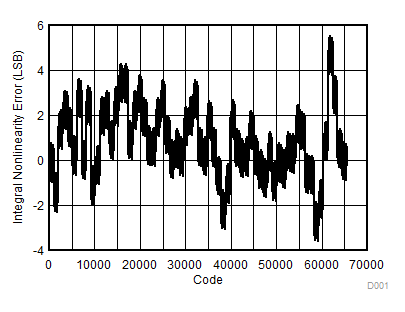Figure 1. Integral Nonlinearity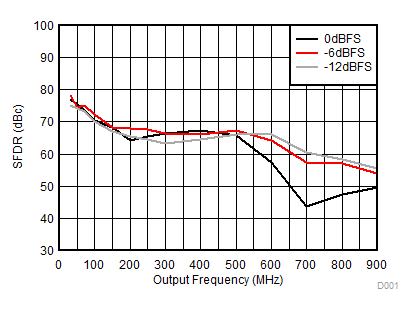Figure 3. SFDR vs Output Frequency Over Input Scale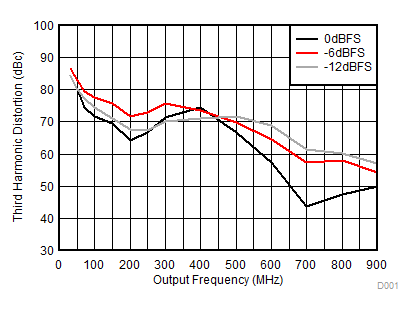Figure 5. Third Harmonic Distortion vs Output Frequency Over Input Scale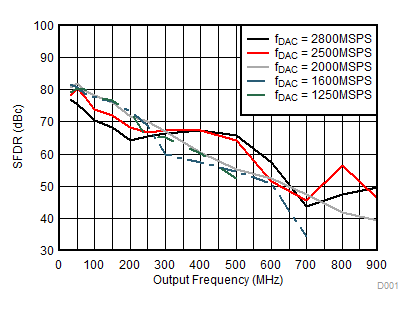4x interpolation for fdac = 2800 msps, 2x interpolation for other cases.
Figure 7. SFDR vs Output Frequency Over fDAC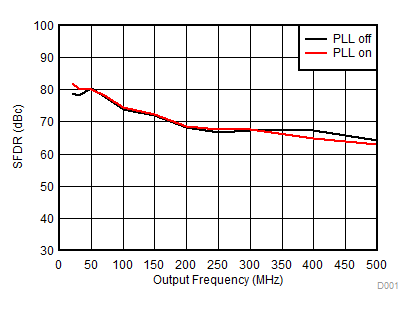fref = fDAC/4, M = 32, N = 8, Prescaler = 2 for PLL On, fDAC=2500MSPS, 2x interpolation.
Figure 9. SFDR vs Output Frequency Over Clocking Options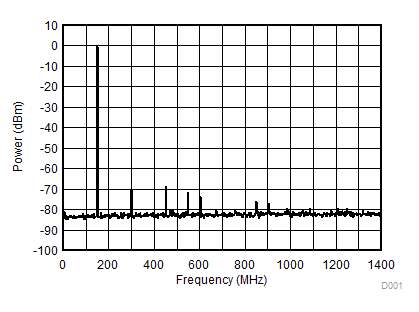IF = 150MHz
Figure 11. Single Tone Spectral Plot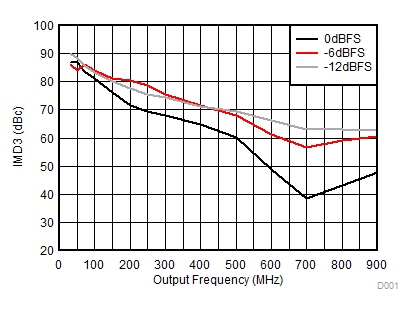A.
Figure 13. IMD3 vs Output Frequency Over Input Scale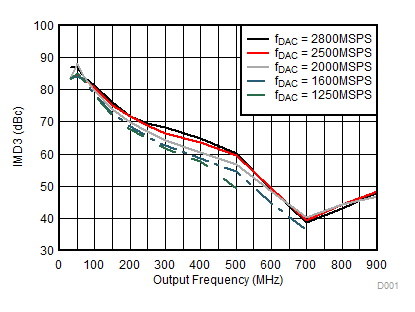4x interpolation for fdac = 2800 msps, 2x interpolation for other cases.
Figure 15. IMD3 vs Output Frequency Over fDAC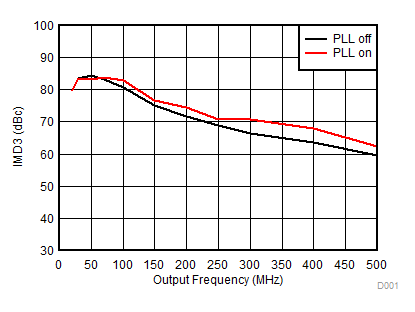fref = fDAC/4, M = 32, N = 8, Prescaler = 2 for PLL On, fDAC=2500MSPS, 2x interpolation.
Figure 17. IMD3 vs Output Frequency Over Clocking Options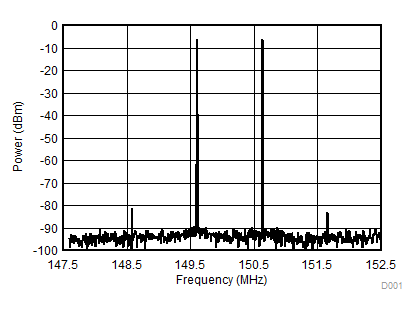IF = 150MHz, Tone Spacing = 1MHz
Figure 19. Two-tone Spectral Plot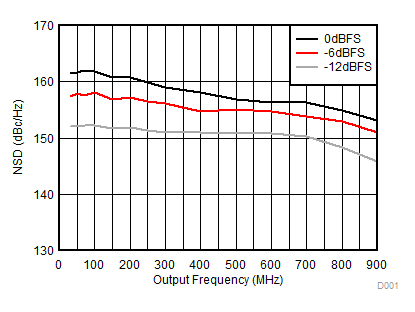Figure 21. NSD vs Output Frequency Over Input Scale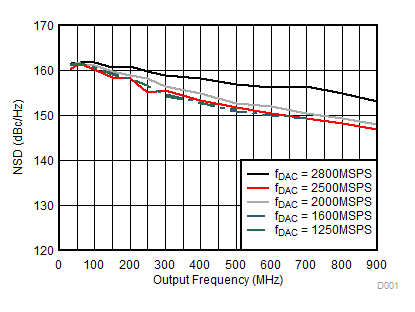4x interpolation for fdac = 2800 msps, 2x interpolation for other cases.
Figure 23. NSD vs Output Frequency Over fDAC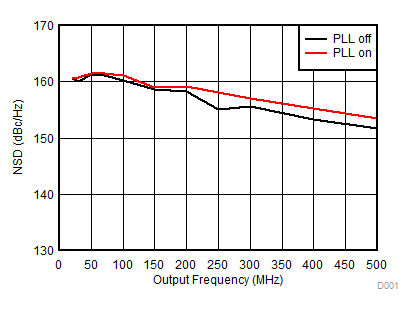fref = fDAC/4, M = 32, N = 8, Prescaler = 2 for PLL On, fDAC=2500MSPS, 2x interpolation.
Figure 25. NSD vs Output Frequency Over Clocking Options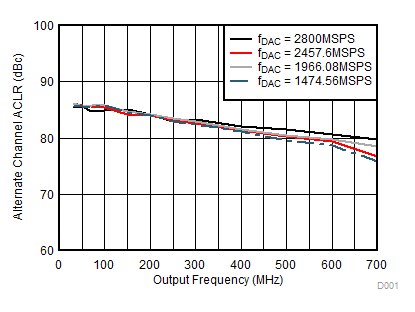Single Carrier WCDMA
Figure 27. ACLR (Alternate Channel) vs Output Frequency Over fDAC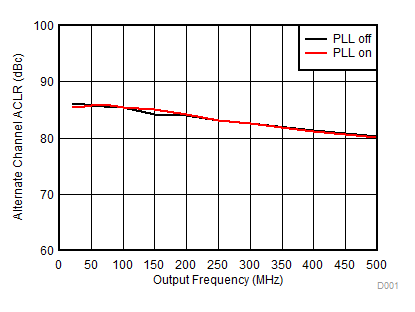Single Carrier WCDMA; fref = fDAC/4, M = 32, N = 8, Prescaler = 2 for PLL On, fDAC=2500MSPS, 2x interpolation.
Figure 29. ACLR (Alternate Channel) vs Output Frequency Over Clocking Options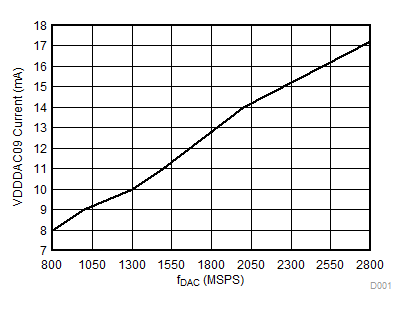Figure 31. VDDDAC Current vs fDAC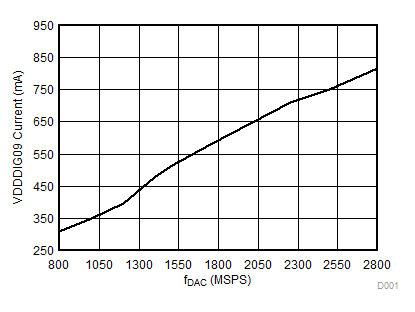Figure 33. VDDDIG Current vs fDAC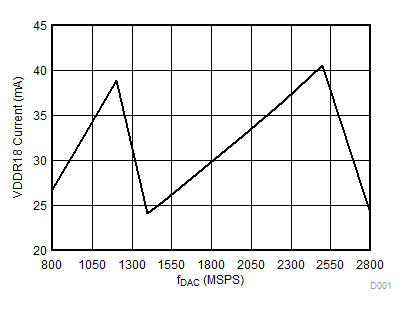Figure 35. VDDR Current vs fDACFigure 37. 1.8V Supply Current Excluding VDDR vs fDAC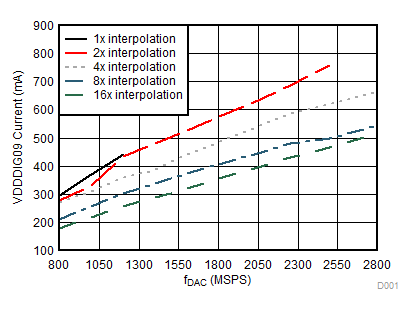QMC Off, CMIX Off, NCO Off, LMF=442 for 16x interpolation
Figure 39. VDDDIG Current vs fDAC Over Interpolation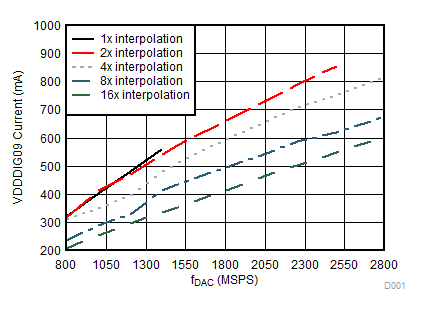QMC On, CMIX On, NCO On, LMF=442 for 16x interpolation
Figure 41. VDDDIG Current vs fDAC Over Interpolation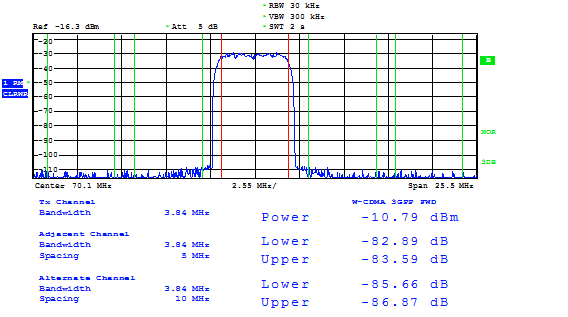IF = 70MHz, fDAC=2457.6MSPS
Figure 43. Single Carrier W-CDMA Test Mode 1IF = 230MHz, fDAC=2457.6MSPS
Figure 45. Single Carrier W-CDMA Test Mode 1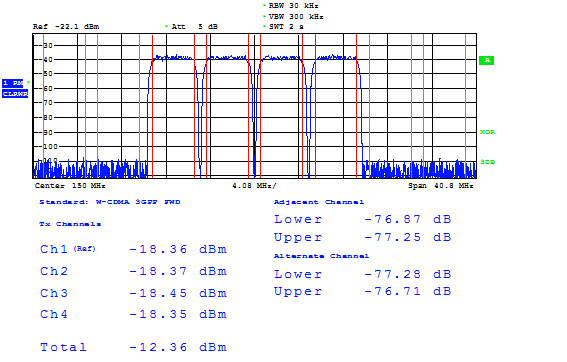IF = 150MHz, fDAC=2457.6MSPS
Figure 47. Four Carrier W-CDMA Test Mode 1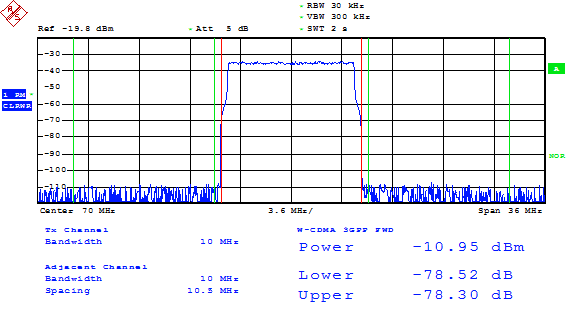IF = 70MHz, fDAC=2457.6MSPS
Figure 49. 10MHz Single Carrier LTE Test Mode 3.1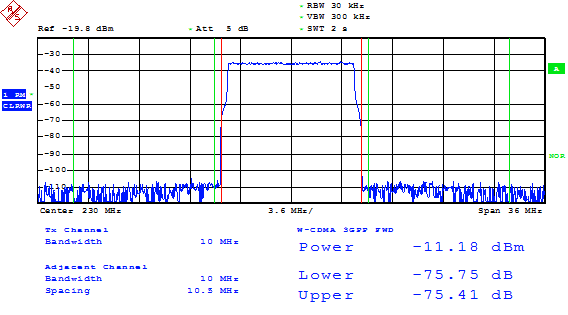IF = 230MHz, fDAC=2457.6MSPS
Figure 51. 10MHz Single Carrier LTE Test Mode 3.1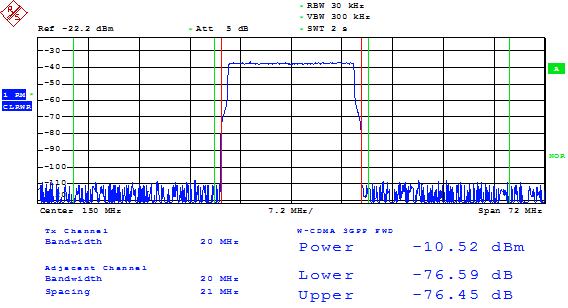IF = 150MHz, fDAC=2457.6MSPS
Figure 53. 20MHz Single Carrier LTE Test Mode 3.1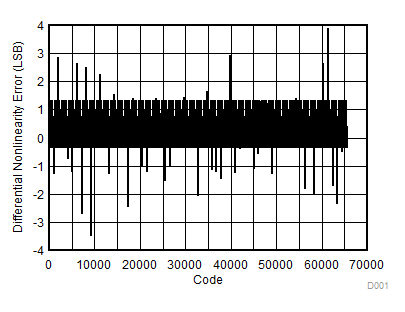Figure 2. Differential Nonlinearity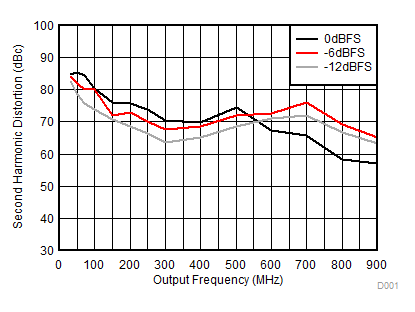Figure 4. Second Harmonic Distortion vs Output Frequency Over Input Scale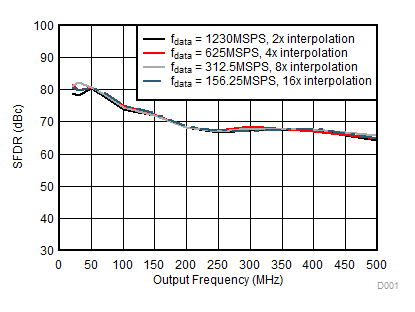Figure 6. SFDR vs Output Frequency Over InterpolationFigure 8. SFDR vs Output Frequency Over IoutFS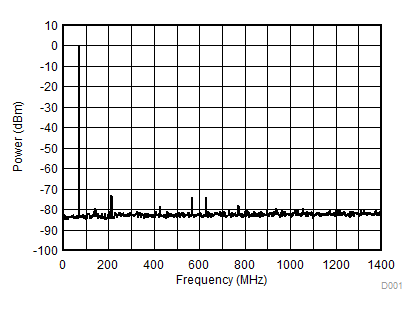IF = 70MHz
Figure 10. Single Tone Spectral Plot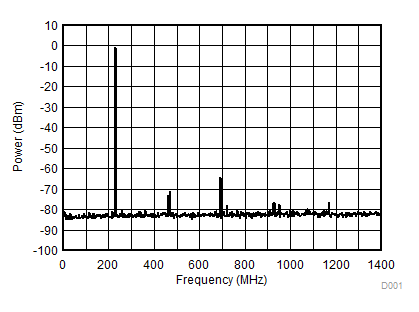IF = 230MHz
Figure 12. Single Tone Spectral Plot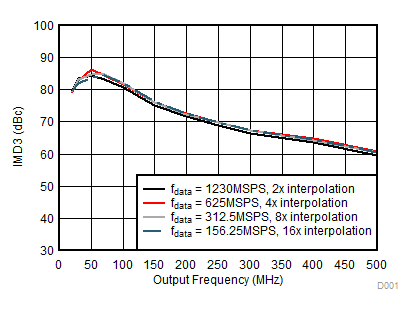Figure 14. IMD3 vs Output Frequency Over Interpolation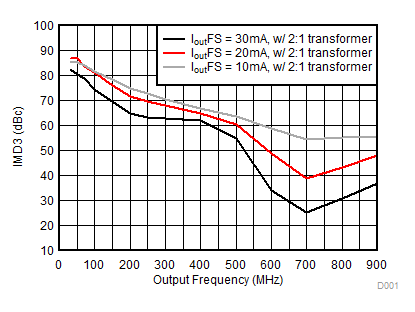Figure 16. IMD3 vs Output Frequency Over Output Current IoutFS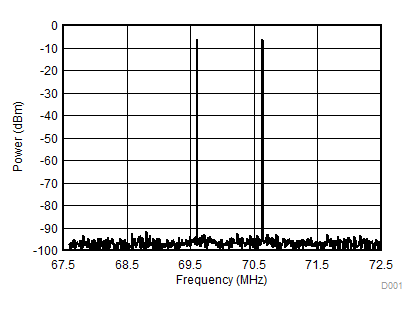IF = 70MHz, Tone Spacing = 1MHz
Figure 18. Two-tone Spectral Plot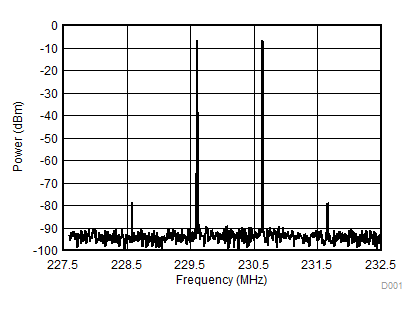IF = 230MHz, Tone Spacing = 1MHz
Figure 20. Two-tone Spectral Plot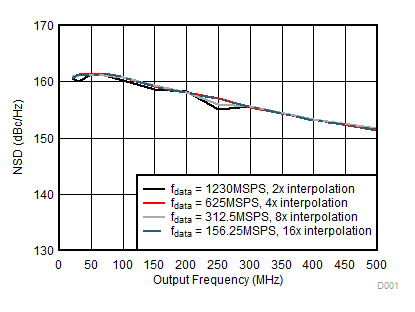Figure 22. NSD vs Output Frequency Over InterpolationFigure 24. NSD vs Output Frequency Over Output Current IoutFS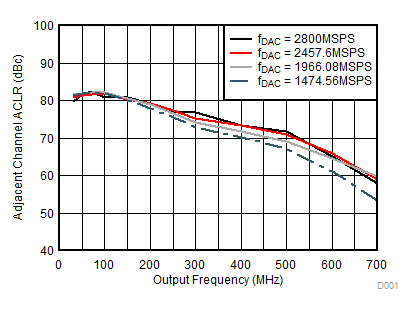Single Carrier WCDMA
Figure 26. ACLR (Adjacent Channel) vs Output Frequency Over fDAC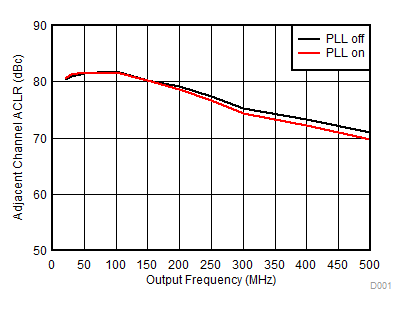Single Carrier WCDMA; fref = fDAC/4, M = 32, N = 8, Prescaler = 2 for PLL On, fDAC=2500MSPS, 2x interpolation.
Figure 28. ACLR (Adjacent Channel) vs Output Frequency Over Clocking Options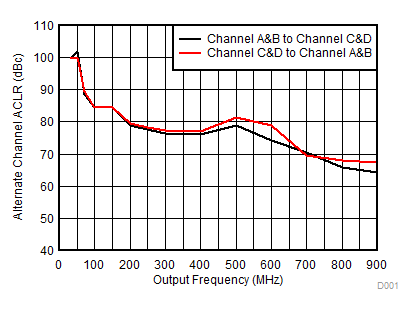Between Channel AB pair and CD pair
Figure 30. Channel Isolation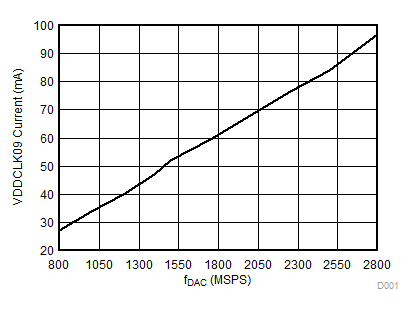Figure 32. VDDCLK Current vs fDAC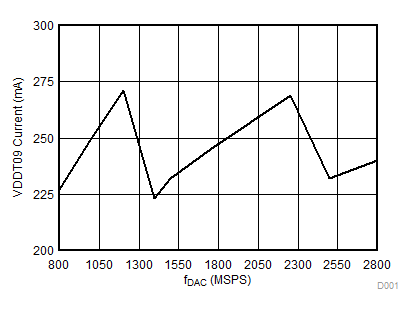Figure 34. VDDT Current vs fDAC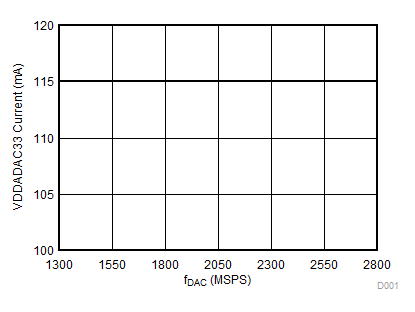Figure 36. VDDADAC Current vs fDACFigure 38. VDDDIG Current vs fDAC Over Digital Processing Functions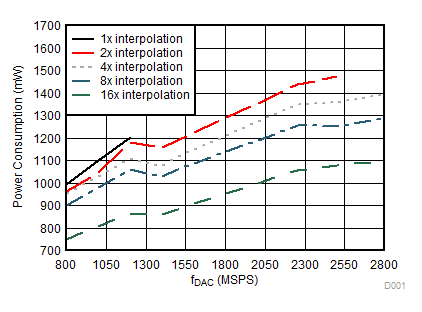QMC Off, CMIX Off, NCO Off, LMF=442 for 16x interpolation
Figure 40. Power Consumption vs fDAC Over Interpolation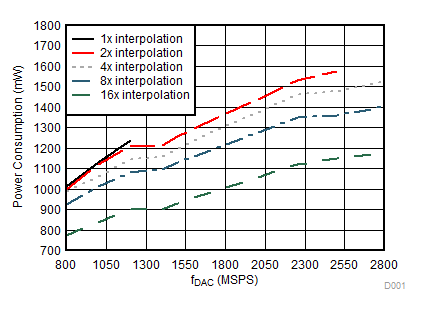QMC On, CMIX On, NCO On, LMF=442 for 16x interpolation
Figure 42. Power Consumption vs fDAC Over Interpolation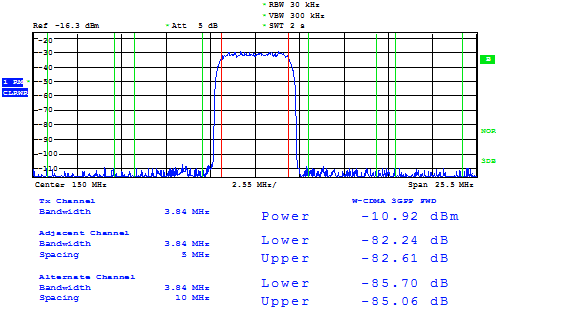IF = 150MHz, fDAC=2457.6MSPS
Figure 44. Single Carrier W-CDMA Test Mode 1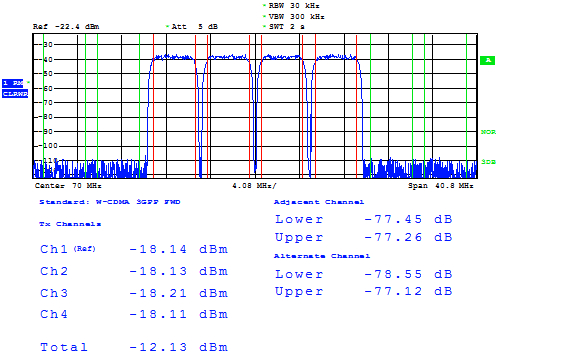IF = 70MHz, fDAC=2457.6MSPS
Figure 46. Four Carrier W-CDMA Test Mode 1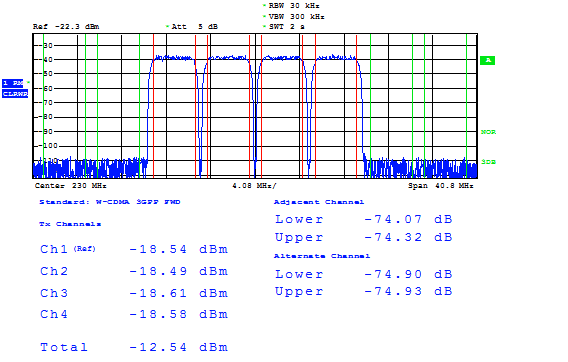IF = 230MHz, fDAC=2457.6MSPS
Figure 48. Four Carrier W-CDMA Test Mode 1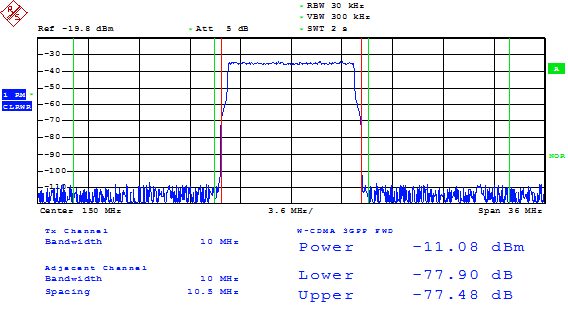IF = 150MHz, fDAC=2457.6MSPS
Figure 50. 10MHz Single Carrier LTE Test Mode 3.1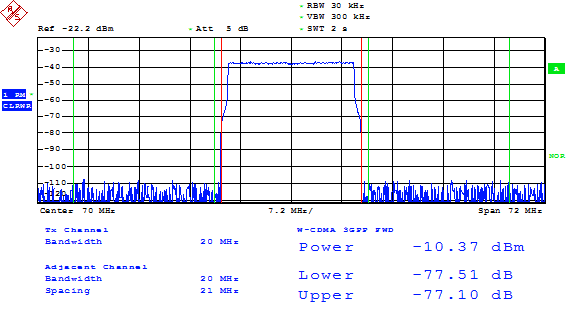IF = 70MHz, fDAC=2457.6MSPS
Figure 52. 20MHz Single Carrier LTE Test Mode 3.1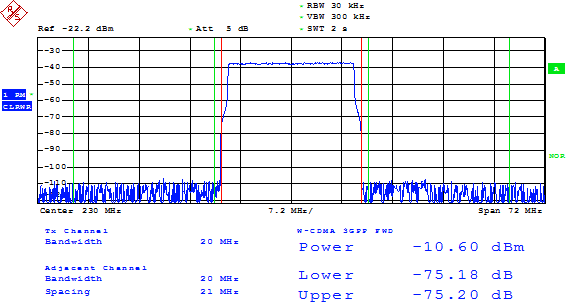IF = 230MHz, fDAC=2457.6MSPS
Figure 54. 20MHz Single Carrier LTE Test Mode 3.1